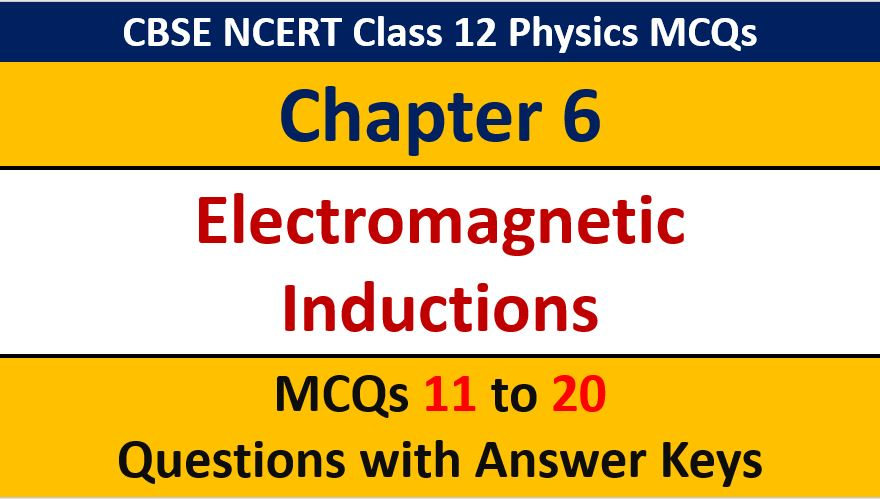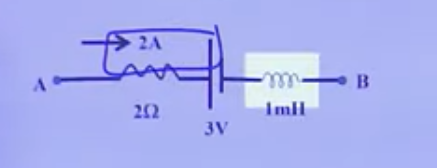## Electromagnetic Induction CBSE Class 12 Physics MCQ Question with Answer Keys Solutions

If you are preparing for CBSE Class 12 Board Exam Term 1 which will be an objective type questions paper, then you need to practice multiple choice questions of Class 12 Physics Chapterwise. In this article of AKVTutorials, you will get Electromagnetic Induction CBSE Class 12 Physics MCQ Question with Answer Keys Solutions

MCQ Questions for Physics Electromagnetic Induction Question No 11:

The flux linked with a coil at any instant ‘t’ is given by, ⧞ = 10t– 50t + 250. The induced emf at t= 3sec is;

Option A : -190 V

Option B : -10 V

Option C : 10 V

Option D : 190

Option B : -10 V

MCQ Questions for Physics Electromagnetic Induction Question No 12:

If ‘N’ is the number of turns in a coil, the value of self inductance varies as

Option A : N0

Option B : N

Option C : N2

Option D : N-2

Option C : N2

MCQ Questions for Physics Electromagnetic Induction Question No 13:

Statement Ⅰ : An inductor in a D.C, Circuit opposes both a steady current and a changing current

Statement Ⅱ : Induced emf is generated only when the flux linked with the induced remains changes.

Option A : Statement Ⅰ is true, Statement Ⅱ is true, Statement Ⅱ is the correct explanation Statement Ⅰ

Option B : Statement Ⅰ is true, Statement Ⅱ is true, Statement Ⅱ is not correct explanation for Student Ⅰ

Option C : Statement Ⅰ is true, Statement Ⅱ is false

Option D : Statement Ⅰ is false, Statement Ⅱ is true

Option D : Statement Ⅰ is false, Statement Ⅱ is true

MCQ Questions for Physics Electromagnetic Induction Question No 14:

A circular coil of ‘a’ turns is kept in a uniform magnetic field such that the plane of the coil is perpendicular to the field. The magnetic flux associated with the coil is now ⧞.Now the coil is opened and made into another circular coil of twice the radius of the previous coil and kept in the same field such that the plane of the coil is perpendicular to the field. The magnetic flux associated with this coil now is

Option A :

Option B : 2

Option C : ⧞ / 4

Option D : ⧞ / 2

Option B : 2

MCQ Questions for Physics Electromagnetic Induction Question No 15:

Statement Ⅰ : The possibility of an electric bulb fusing is higher at the time of switching on and off

Statement Ⅱ : Inductive effects produce  a large current at the time of switch-on and switch-off

Option A : Statement Ⅰ is true, Statement Ⅱ is true, Statement is the correct explanation for Statement Ⅰ.

Option B : Statement Ⅰ is true, Statement Ⅱ is true, statement Ⅱ is not correct explanation for Statement Ⅰ

Option C : Statement Ⅰ is true, Statement Ⅱ is false

Option D : Statement Ⅰ is false, Statement Ⅱ is true

Option A : Statement Ⅰ is true, Statement Ⅱ is true, Statement is the correct explanation for Statement Ⅰ.

MCQ Questions for Physics Electromagnetic Induction Question No 16:

A flux of 8.66 milli weber passes through a strip having an area A= 0.02 m2. The plane of the strip is at n angle of 60to the direction of the magnetic field B. The value of B is

Option A : 0.5 T

Option B : 0.866 T

Option C : 0.25 T

Option D : 0.433 T

Option A : 0.5 T

MCQ Questions for Physics Electromagnetic Induction Question No 17:

In a magnetic field of induction 0.9 weber of 0.4 meter length is moving with a velocity of 7 m/s. The value of maximum induced emf will be (in weber / m2)

Option A : 2.52 volt

Option B : 5.26 volt

Option C : 6.28 volt

Option D : 0.26 volt

Option A : 2.52 volt

MCQ Questions for Physics Electromagnetic Induction Question No 18:

A wheel has three spokes and is in a uniform magnetic field perpendicular to its plane, with the axis of rotation of the wheel parallel to the magnetic field. When the wheel rotates with a uniform angular velocity ω , the emf induced between the center and rim of the wheel is ‘e’. If another wheel having same radius but with six spokes is kept in the same field and rotated with a uniform angular velocity ‘ω / 2’, the emf induced between  the center and the rim will be

Option A : e

Option B : e/2

Option C : 2e

Option D : e / 4

Option B : e/2

MCQ Questions for Physics Electromagnetic Induction Question No 19:

If current is decreasing at a rate of 1000 As-1 , p.d. between A and B isOption A : 6 V

Option B : 5V

Option C : 7 V

Option D : 8 V

Option D : 8 V

MCQ Questions for Physics Electromagnetic Induction Question No 20:

An average emf of 32 V is induced in a coil in a coil in which the current drops from 10A to 2A  in 0.1s. The inductance of the coil is

Option A : 0.32 H

Option B : 0.4 H

Option C : 4 H

Option D : 0.04 H

Option B : 0.4 H

CBSE Class 12 Physics Chapterwise MCQ
1 : Electric Charge & Fields MCQs
| 1 to 10 | 11 to 20
2 : Electrostatics Potential and Capacitance MCQs
| 1 to 10 | 11 to 20
3 : Current Electricity MCQs
| 1 to 10 | 11 to 20
4 : Moving charges and Magnetism MCQs
| 1 to 10 | 11 to 20
5 : Magnetism and Matter MCQs
| 1 to 10 | 11 to 20
6 : Electromagnetic Induction MCQs
| 1 to 10 | 11 to 20
7 : Alternating Current MCQs
| 1 to 10 | 11 to 20
8 : Electromagnetic Waves MCQs
| 1 to 10 | 11 to 20
9 : Ray Optics and Optical Instruments MCQs
| 1 to 10 | 11 to 20
10 : Wave Optics MCQs
| 1 to 10 | 11 to 20
11 : Dual Nature of Radiation MCQs
| 1 to 10 | 11 to 20
12 : Atoms MCQs
| 1 to 10 | 11 to 20
13 : Nuclei MCQs
| 1 to 10 | 11 to 20
14 : Semi Conductor Electronics MCQs
| 1 to 10 | 11 to 20
15 : Communication Systems MCQs
| 1 to 10 | 11 to 20